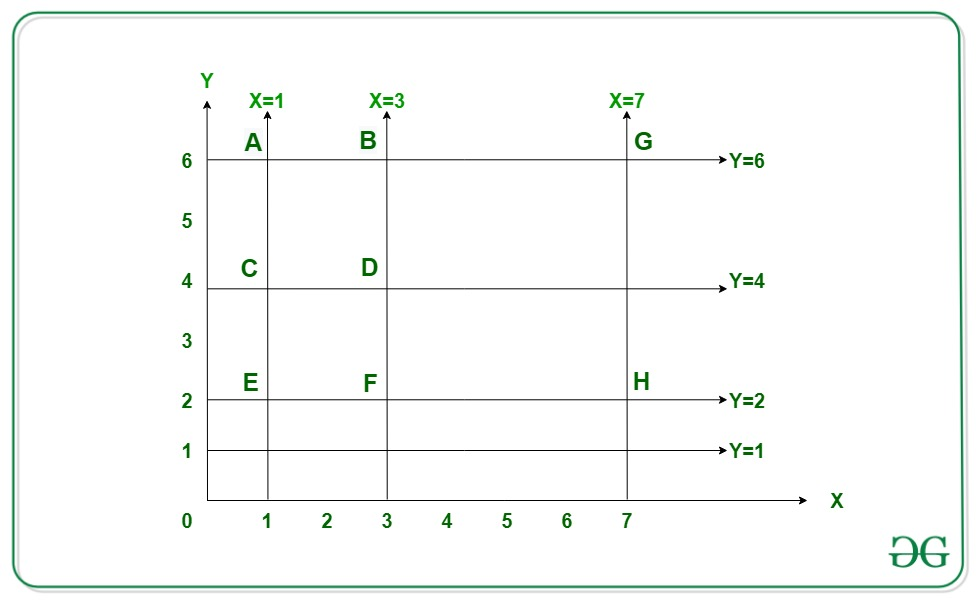# Count squares of unique dimensions possible from given Straight Lines parallel to the axes

Given two arrays X[] and Y[] consisting of N and M integers such that there are N lines parallel to the y-axis and M lines parallel to the x-axis, the task is to find the total number of squares having unique dimensions that can be generated from the given straight lines.

Each integer(say a) in the array X[] denotes lines having equation x = a, parallel to
y-axis
Each integer(say b) in the array Y[] denotes lines having equation y = b, parallel to
x-axis

Examples:

Input: X[] = {1, 3, 7}, Y[] = {1, 2, 4, 6}
Output:
Explanation:
3 lines are parallel to y-axis for x = 1, x = 3 and x = 7.
4 lines are parallel to x-axis for y = 2, y = 4, y = 6 and y = 1.From the above figure, there are two possible squares of unique dimensions that are possible:
1) square ABDC (x = 1, x = 3, y = 4, y = 6), side = 2 units.
2) square BGHF (x = 3, x = 7, y = 2, y = 6), side = 4 units.

Input: X[] = {2, 6, 7, 8}, Y[] = {1, 3, 5, 7}
Output: 3

Naive Approach: The simplest approach is to check for every possible vertical dimension if there exists an equal horizontal dimension. The time complexity of this approach can be reduced with the help of the map. By using a map, we can get all the different vertical/horizontal dimensions.
i>Time Complexity: O((N2)*log N)
Auxiliary Space: O(N)

Efficient Approach: To optimize the above approach, the idea is to generate all possible dimensions with the help of bitsets. Follow the steps below to solve this problem:

• Initialize bitsets for horizontal and vertical lines from the array X[] and Y[] respectively.
• Set positions of vertical and horizontal lines in the bitset.
• Maintain another bitset hdiff and vdiff that store the different possible dimensions of squares. The dimensions can be obtained by shifting the set bits in the original bitset.
• After the above steps, the unique count of squares is the count of the common element in hdiff and vdiff which is calculated by (hdiff&vdiff).count().

Below is the implementation of the above approach:

## C++

 `// C++ program for the above approach ` ` `  `#include ` `using` `namespace` `std; ` `const` `int` `N = 100; ` ` `  `// Function to find the number of ` `// unique squares ` `int` `countSquares(``int``* hor, ``int``* ver, ` `                 ``int` `n, ``int` `m) ` `{ ` `    ``// Positions of the X[] and Y[] ` `    ``// are set in the bitsets hpos ` `    ``// and vpos respectively ` `    ``bitset hpos, vpos; ` ` `  `    ``for` `(``int` `i = 0; i < n; ++i) { ` `        ``hpos.set(hor[i]); ` `    ``} ` `    ``for` `(``int` `i = 0; i < m; ++i) { ` `        ``vpos.set(ver[i]); ` `    ``} ` ` `  `    ``// Stores all possible sides of the ` `    ``// square are set in the bitsets ` `    ``// having difference hdiff & vdiff ` `    ``bitset hdiff, vdiff; ` ` `  `    ``for` `(``int` `i = 0; i < n; ++i) { ` `        ``hdiff = hdiff | (hpos >> hor[i]); ` `    ``} ` `    ``for` `(``int` `i = 0; i < m; ++i) { ` `        ``vdiff = vdiff | (vpos >> ver[i]); ` `    ``} ` ` `  `    ``// Finding the number of square ` `    ``// sides which are common to both ` `    ``int` `common = (hdiff & vdiff).count(); ` ` `  `    ``// Print the count of squares ` `    ``cout << common - 1; ` `} ` ` `  `// Driver Code ` `int` `main() ` `{ ` `    ``// Given horizontal line segments ` `    ``int` `X[] = { 1, 3, 7 }; ` ` `  `    ``// Given vertical line segments ` `    ``int` `Y[] = { 1, 2, 4, 6 }; ` ` `  `    ``int` `N = (``sizeof``(X) / ``sizeof``(X)); ` `    ``int` `M = (``sizeof``(Y) / ``sizeof``(Y)); ` ` `  `    ``// Function Call ` `    ``countSquares(X, Y, N, M); ` ` `  `    ``return` `0; ` `} `

Output:

```2
```

Time Complexity: O(N + M)
Auxiliary Space: O(maxE), where maxE is the maximum element among both the arrays X[] and Y[].

Attention reader! Don’t stop learning now. Get hold of all the important DSA concepts with the DSA Self Paced Course at a student-friendly price and become industry ready.

My Personal Notes arrow_drop_upCheck out this Author's contributed articles.

If you like GeeksforGeeks and would like to contribute, you can also write an article using contribute.geeksforgeeks.org or mail your article to contribute@geeksforgeeks.org. See your article appearing on the GeeksforGeeks main page and help other Geeks.

Please Improve this article if you find anything incorrect by clicking on the "Improve Article" button below.